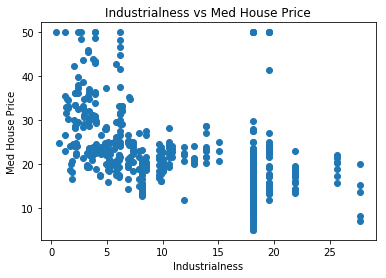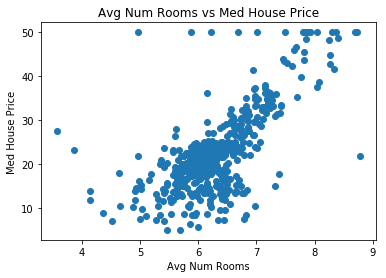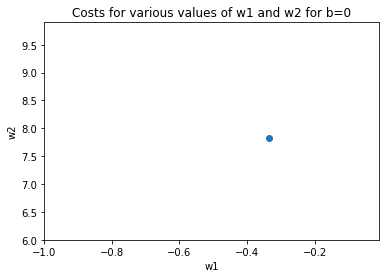# CSC321 Tutorial 2: Linear Regression¶

In this tutorial, we'll go through another example of linear regression from an implementation perspective. We will use the Boston Housing dataset, and predict the median cost of a home in an area of Boston. We will:

• set up the linear regression problem using numpy
• show that vectorized code is faster (more in Lecture 2)
• solve the linear regression problem using the closed form solution
• solve the linear regression problem using gradient descent (more in Lecture 2)
In :
import matplotlib
import numpy as np
import matplotlib.pyplot as plt
%matplotlib inline


## Boston Housing Data¶

The Boston Housing data is one of the "toy datasets" available in sklearn. We can import and display the dataset description like this:

In :
from sklearn.datasets import load_boston
print(boston_data['DESCR'])

.. _boston_dataset:

Boston house prices dataset
---------------------------

**Data Set Characteristics:**

:Number of Instances: 506

:Number of Attributes: 13 numeric/categorical predictive. Median Value (attribute 14) is usually the target.

:Attribute Information (in order):
- CRIM     per capita crime rate by town
- ZN       proportion of residential land zoned for lots over 25,000 sq.ft.
- INDUS    proportion of non-retail business acres per town
- CHAS     Charles River dummy variable (= 1 if tract bounds river; 0 otherwise)
- NOX      nitric oxides concentration (parts per 10 million)
- RM       average number of rooms per dwelling
- AGE      proportion of owner-occupied units built prior to 1940
- DIS      weighted distances to five Boston employment centres
- RAD      index of accessibility to radial highways
- TAX      full-value property-tax rate per $10,000 - PTRATIO pupil-teacher ratio by town - B 1000(Bk - 0.63)^2 where Bk is the proportion of blacks by town - LSTAT % lower status of the population - MEDV Median value of owner-occupied homes in$1000's

:Missing Attribute Values: None

:Creator: Harrison, D. and Rubinfeld, D.L.

This is a copy of UCI ML housing dataset.
https://archive.ics.uci.edu/ml/machine-learning-databases/housing/

This dataset was taken from the StatLib library which is maintained at Carnegie Mellon University.

The Boston house-price data of Harrison, D. and Rubinfeld, D.L. 'Hedonic
prices and the demand for clean air', J. Environ. Economics & Management,
vol.5, 81-102, 1978.   Used in Belsley, Kuh & Welsch, 'Regression diagnostics
...', Wiley, 1980.   N.B. Various transformations are used in the table on
pages 244-261 of the latter.

The Boston house-price data has been used in many machine learning papers that address regression
problems.

.. topic:: References

- Belsley, Kuh & Welsch, 'Regression diagnostics: Identifying Influential Data and Sources of Collinearity', Wiley, 1980. 244-261.
- Quinlan,R. (1993). Combining Instance-Based and Model-Based Learning. In Proceedings on the Tenth International Conference of Machine Learning, 236-243, University of Massachusetts, Amherst. Morgan Kaufmann.



To keep the example simple, we will only work with two features: INDUS and RM. The explanations of these features are in the description above.

In :
# take the boston data
data = boston_data['data']
# we will only work with two of the features: INDUS and RM
x_input = data[:, [2,5]]
y_target = boston_data['target']


Just to give us an intuition of how these two features INDUS and RM affect housing prices, lets visualize the feature interactions. As expected, the more "industrial" a neighbourhood is, the lower the housing prices. The more rooms houses in a neighbourhood have, the higher the median housing price.

In :
# Individual plots for the two features:
plt.title('Industrialness vs Med House Price')
plt.scatter(x_input[:, 0], y_target)
plt.xlabel('Industrialness')
plt.ylabel('Med House Price')
plt.show()

plt.title('Avg Num Rooms vs Med House Price')
plt.scatter(x_input[:, 1], y_target)
plt.xlabel('Avg Num Rooms')
plt.ylabel('Med House Price')
plt.show()## Defining the Cost Function¶

In lecture, we defined the cost function for a linear regression problem using the square loss:

$$\mathcal{E}(y, t) = \frac{1}{2N} \sum_{i=1}^N (y^{(i)}-t^{(i)})^2$$

In our case, since we have two features $x_1$ and $x_2$, our linear regression model will look like this:

$$\mathcal{E}(y, t) = \frac{1}{2N} \sum_{i=1}^N (w_1 x_1^{(i)} + w_2 x_2^{(i)} + b -t^{(i)})^2$$

We can use the above formula to compute the cost function across an entire dataset (X, t):

In :
def cost(w1, w2, b, X, t):
'''
Evaluate the cost function in a non-vectorized manner for
inputs X and targets t, at weights w1, w2 and b.
'''
# TODO: write this!


For example, the cost for this hypothesis...

In :
cost(3, 5, 20, x_input, y_target)


...is higher than this one:

In :
cost(3, 5, 0, x_input, y_target)


## Vectorizing the cost function:¶

Vectorization is a way to use linear algbera to represent computations like the one above. In Python, vectorized code written in numpy tend to be faster than code that uses a for loop. We'll talk about vectorization in more detail in lecture 2.

If we write the linear regression cost function using matrix computations, it would look like this:

$$\mathcal{E}(y, t) = \frac{1}{2N} \| \bf{X} \bf{w} + b \bf{1} - \bf{t} \| ^2$$

Following the above formula, our vectorized code looks like this:

In :
def cost_vectorized(w1, w2, b, X, t):
'''
Evaluate the cost function in a vectorized manner for
inputs X and targets t, at weights w1, w2 and b.
'''
# TODO: write this!


We can check that the vectorized code provides the same answers as the non-vectorized code:

In :
# TODO: write this!

In :
# TODO: write this!


## Comparing speed of the vectorized vs unvectorized code¶

We'll see below that the vectorized code already runs ~2x faster than the non-vectorized code!

Hopefully this will convince you to always vectorized your code whenever possible

In :
import time


Time for non-vectorized code:

In :
t0 = time.time()
print(cost(4, 5, 20, x_input, y_target))
t1 = time.time()
print(t1 - t0)

None
0.0002777576446533203


Time for vectorized code:

In :
t0 = time.time()
print(cost_vectorized(4, 5, 20, x_input, y_target))
t1 = time.time()
print(t1 - t0)

None
0.0005860328674316406


## Plotting cost in weight space¶

We'll plot the cost for two of our weights, assuming that bias = -22.89831573.

We'll see where that number comes from later.

Notice the shape of the contours are ovals.

In :
w1s = np.arange(-1.0, 0.0, 0.01)
w2s = np.arange(6.0, 10.0, 0.1)
z_cost = []
for w2 in w2s:
z_cost.append([cost_vectorized(w1, w2, -22.89831573, x_input, y_target) for w1 in w1s])
z_cost = np.array(z_cost)
np.shape(z_cost)
W1, W2 = np.meshgrid(w1s, w2s)
CS = plt.contour(W1, W2, z_cost, 25)
plt.clabel(CS, inline=1, fontsize=10)
plt.title('Costs for various values of w1 and w2 for b=0')
plt.xlabel("w1")
plt.ylabel("w2")
plt.plot([-0.33471389], [7.82205511], 'o') # this will be the minima that we'll find later
plt.show()

/Users/xuexue/miniconda3/lib/python3.6/site-packages/matplotlib/contour.py:1557: UserWarning: Warning: converting a masked element to nan.
self.zmax = float(z.max())
/Users/xuexue/miniconda3/lib/python3.6/site-packages/matplotlib/contour.py:1558: UserWarning: Warning: converting a masked element to nan.
self.zmin = float(z.min())
/Users/xuexue/miniconda3/lib/python3.6/site-packages/matplotlib/contour.py:1203: RuntimeWarning: invalid value encountered in less
under = np.nonzero(lev < self.zmin)
/Users/xuexue/miniconda3/lib/python3.6/site-packages/matplotlib/contour.py:1205: RuntimeWarning: invalid value encountered in greater
over = np.nonzero(lev > self.zmax)
/Users/xuexue/miniconda3/lib/python3.6/site-packages/matplotlib/contour.py:1239: RuntimeWarning: invalid value encountered in greater
inside = (self.levels > self.zmin) & (self.levels < self.zmax)
/Users/xuexue/miniconda3/lib/python3.6/site-packages/matplotlib/contour.py:1239: RuntimeWarning: invalid value encountered in less
inside = (self.levels > self.zmin) & (self.levels < self.zmax)
/Users/xuexue/miniconda3/lib/python3.6/site-packages/matplotlib/contour.py:1243: UserWarning: No contour levels were found within the data range.
warnings.warn("No contour levels were found"## Exact Solution¶

Work this out on the board:

1. ignore biases (add an extra feature & weight instead)
2. get equations from partial derivative
3. vectorize
4. write code.
In :
# add an extra feature (column in the input) that are just all ones
x_in = np.concatenate([x_input, np.ones([np.shape(x_input), 1])], axis=1)
x_in

Out:
array([[ 2.31 ,  6.575,  1.   ],
[ 7.07 ,  6.421,  1.   ],
[ 7.07 ,  7.185,  1.   ],
...,
[11.93 ,  6.976,  1.   ],
[11.93 ,  6.794,  1.   ],
[11.93 ,  6.03 ,  1.   ]])
In :
def solve_exactly(X, t):
'''
Solve linear regression exactly. (fully vectorized)

Given X - NxD matrix of inputs
t - target outputs
Returns the optimal weights as a D-dimensional vector
'''
# TODO: write this!

In :
solve_exactly(x_in, y_target)


In practice, we use library code that is written for us.

In :
# In real life we don't want to code it directly
np.linalg.lstsq(x_in, y_target)

/Users/xuexue/miniconda3/lib/python3.6/site-packages/ipykernel_launcher.py:2: FutureWarning: rcond parameter will change to the default of machine precision times max(M, N) where M and N are the input matrix dimensions.
To use the future default and silence this warning we advise to pass rcond=None, to keep using the old, explicitly pass rcond=-1.


Out:
(array([ -0.33471389,   7.82205511, -22.89831573]),
array([19807.614505]),
3,
array([318.75354429,  75.21961717,   2.10127199]))

Another approach to optimize the cost function is via gradient descent, which we will discuss in lecture 2.

The main idea is that we compute the gradient of the cost function with respect to each parameter $w_j$, which will tell us how to update $w_j$ (by a small amount) to improve the cost function (by a small amount).

In order to implement gradient descent, we need to be able to compute the gradient of the cost function with respect to a weight $w_j$:

$$\frac{\partial \mathcal{E}}{\partial w_j} = \frac{1}{N}\sum_i x_j^{(i)}(y^{(i)}-t^{(i)})$$

In :
# Vectorized gradient function
def gradfn(weights, X, t):
'''
Given weights - a current "Guess" of what our weights should be
X - matrix of shape (N,D) of input features
t - target y values
Return gradient of each weight evaluated at the current value
'''
# TODO: write this!


With this function, we can solve the optimization problem by repeatedly applying gradient descent on $w$:

In :
def solve_via_gradient_descent(X, t, print_every=5000,
niter=100000, alpha=0.005):
'''
Given X - matrix of shape (N,D) of input features
t - target y values
Solves for linear regression weights.
Return weights after niter iterations.
'''
# TODO: write this!

In :
# TODO: write this!


For comparison, this was the exact result:

In :
np.linalg.lstsq(x_in, y_target)

/Users/xuexue/miniconda3/lib/python3.6/site-packages/ipykernel_launcher.py:1: FutureWarning: rcond parameter will change to the default of machine precision times max(M, N) where M and N are the input matrix dimensions.
To use the future default and silence this warning we advise to pass rcond=None, to keep using the old, explicitly pass rcond=-1.
"""Entry point for launching an IPython kernel.

Out:
(array([ -0.33471389,   7.82205511, -22.89831573]),
array([19807.614505]),
3,
array([318.75354429,  75.21961717,   2.10127199]))